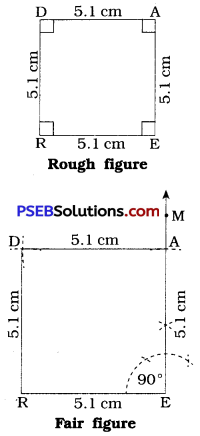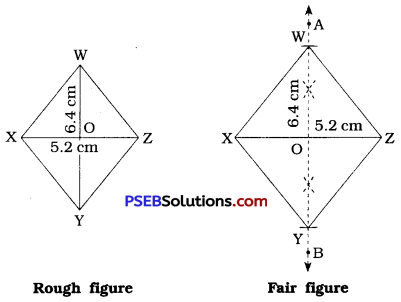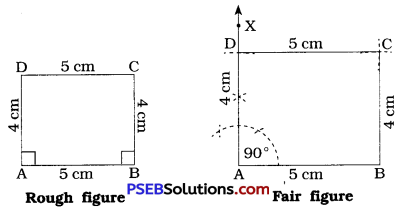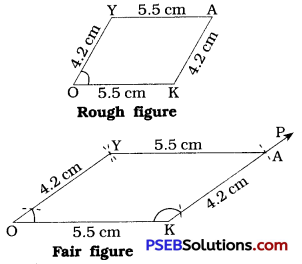# PSEB 8th Class Maths Solutions Chapter 4 Practical Geometry Ex 4.5

Punjab State Board PSEB 8th Class Maths Book Solutions Chapter 4 Practical Geometry Ex 4.5 Textbook Exercise Questions and Answers.

## PSEB Solutions for Class 8 Maths Chapter 4 Practical Geometry Ex 4.5

Draw the following.

Question 1.
The square READ with RE = 5.1 cm.
Solution:Steps of construction :

• Draw a line segment RE = 5.1 cm.
• At E, draw $$\overrightarrow{\mathrm{EM}}$$, such that ∠REM = 90°.
• With E as centre and radius =5.1 cm, draw an arc intersecting $$\overrightarrow{\mathrm{EM}}$$ at A.
• With R as centre and radius = 5.1 cm, draw an arc.
• With A as centre and radius = 5.1 cm, draw an arc which intersect the previous arc at D.
• Draw $$\overline{\mathrm{RD}}$$ and $$\overline{\mathrm{AD}}$$.

Thus, READ is the required square.Question 2.
A rhombus whose diagonals are 5.2 cm and 6.4 cm long.
Solution:[Note : The diagonals of a rhombus bisect each other at right angle.]
Here, in rhombus XYZW, YW 6.4 cm.
∴ OW = OY = 3.2 cm

Steps of construction:

• Draw a line segment XZ = 5.2 cm.
• Draw $$\overleftrightarrow{\mathrm{AB}}$$, the perpendicular bisector of $$\overline{\mathrm{XZ}}$$, which intersect $$\overline{\mathrm{XZ}}$$ at O.
• With O as centre and radius = 3.2 cm, draw an arc intersecting $$\overleftrightarrow{\mathrm{AB}}$$ above $$\overline{\mathrm{XZ}}$$ at W.
• With O as centre and radius = 3.2 cm, draw another arc which intersects $$\overleftrightarrow{\mathrm{AB}}$$ below $$\overline{\mathrm{XZ}}$$ at Y.
• Draw $$\overline{\mathrm{XY}}$$, $$\overline{\mathrm{YZ}}$$, $$\overline{\mathrm{ZW}}$$ and $$\overline{\mathrm{XW}}$$.

Thus, XYZW is the required rhombus.

Question 3.
A rectangle with adjacent sides of lengths 5 cm and 4 cm.
Solution:[Note: Each angle of a rectangle is a right angle.]
Steps of construction :

• Draw a line segment AB = 5 cm.
• At A, draw $$\overrightarrow{\mathrm{AX}}$$, such that ∠XAB = 90°.
• With A as centre and radius = 4 cm, draw an arc intersecting $$\overrightarrow{\mathrm{AX}}$$ at D.
• With B as centre and radius = 4 cm, draw an arc.
• With D as centre and radius = 5 cm, draw an arc which intersects the previous arc at C.
• Draw $$\overline{\mathrm{BC}}$$ and $$\overline{\mathrm{CD}}$$.

Thus, ABCD is the required rectangle.Question 4.
A parallelogram OKAY where OK = 5.5 cm and KA = 4.2 cm. Is it unique ?
Solution:A parallelogram cannot be constructed as sufficient measurements are not given. It is not unique as angles may vary in parallelogram drawn by given measurements.